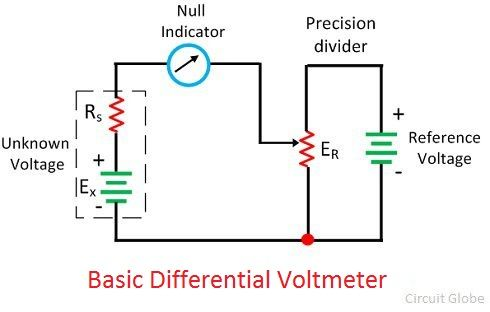# Differential Voltmeter

Definition: The voltmeter which measures the difference between the known and the unknown voltage source is known as the differential voltmeter. It works on the principle of comparison between the reference and the unknown voltage sources.

The accuracy of the differential voltmeter is very high.The principle of operation of the differential voltmeter is similar to that of the potentiometer, and hence it is called potentiometer voltmeter.

## Construction of Differential Voltmeter

The circuit diagram of the differential voltmeter is shown in the figure below. The null meter is placed between the unknown voltage source and the precision divider. The output of the precision divider is connected to the known voltage source. The precision divider is adjusted until the meter shows the zero deflection.The null deflection of the meter shows that the magnitude of both the known and the unknown voltage source becomes equal. During the null deflection, neither the known nor the unknown source applies current to the meter. And the voltmeter shows the high impedance to the measurand source.

The null meter only shows the residual difference between the known and the unknown voltage source. For determining the exact difference between the sources, the more sensitive meter is used.

The low voltage DC standard source or the low voltage Zener controlled precision supply is used as a reference voltage. The high voltage supply is used for measuring the high voltages.

## Types of Differential Voltmeter

The differential voltmeter is of two types.

1. AC Differential Voltmeter
2. DC Differential Voltmeter

### AC Differential Voltmeter

The differential voltmeter is the improved form of the DC instruments. The unknown AC voltage source applies to the rectifier which converts the AC voltage to the DC voltage with equivalent magnitude. The resultant DC apply to the potentiometer for comparing it with the known voltage source. The block diagram of the AC differential voltmeter shown in the figure below.The rectifying AC voltage compares with the standard DC voltage and the meter shows the null deflection when their magnitudes become equals. Thus, the value of unknown voltage is determined.

### DC Differential Voltmeter

The unknown DC source acts as an input to the amplifier section. The fraction of the output voltage is feedback to the input voltage with the help of the one divider network. The other section of the voltage divider supplies the fractional input to the meter amplifier.The meter measures the difference between the feedback voltage and the reference voltage. If the magnitude of both the unknown and reference voltages becomes zero, then the null meter shows the null deflection.